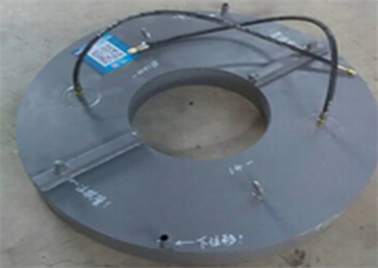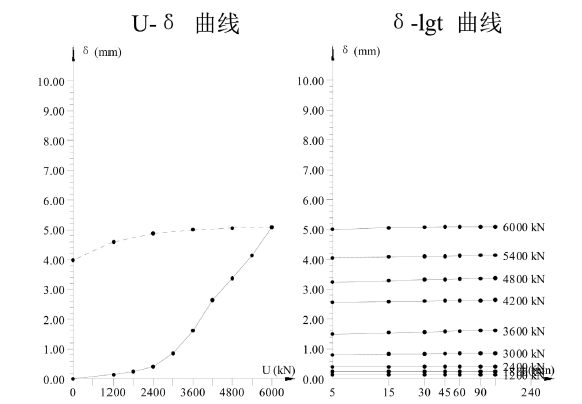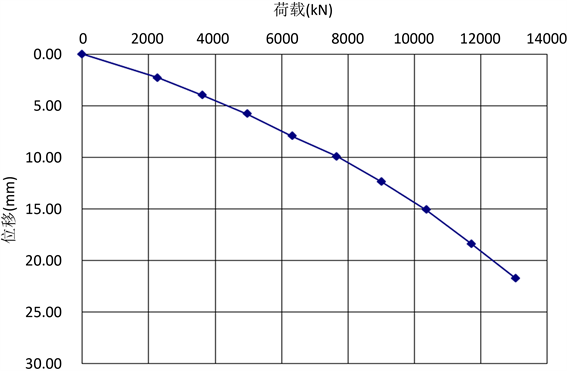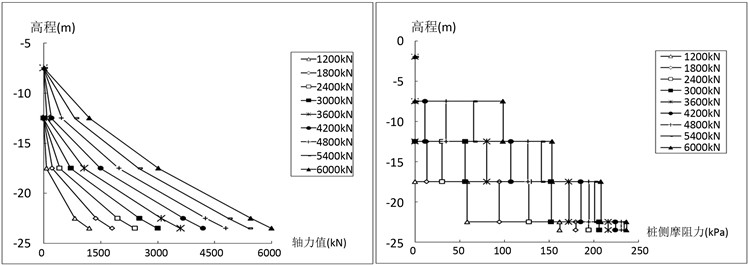﻿ 高速公路改建工程桩基自平衡试验及桩的受力特性研究

# 高速公路改建工程桩基自平衡试验及桩的受力特性研究Study on Self-Balance Test of Pile Foundation and Force Characteristics of Piles in Highway Reconstruction Engineering

Abstract: Compared with the traditional pile loading method or pile method, self-balancing method can save time and effort. It has the characteristics of safety, environmental protection, less occupied space, and a wide range of applications. Especially for large diameter and large tonnage piles, which is difficult and unsafe to test the pile with traditional static load test, the bearing capacity data ob-tained is not accurate enough, and the bearing capacity is wasted. In this case, the advantage of self-balancing method will be more obvious. Based on the reconstruction project of Changsha Liutuo section of the Beijing-Hong Kong-Macao expressway, self-balanced method is used to test the pile axial force and lateral friction force of the large tonnage pile, which can determine the actual bearing capacity and test the difference of the measured friction force and side friction resistance in survey , and judge the bearing capacity of pile under the same geological condition.

1. 引言Table 1. The main parameter of test pile

2. 概况

2.1. 工程概况

2.2. 工程地质条件

3. 设备及元器件埋设

3.1. 平衡点计算及荷载箱埋设

3.2. 钢筋计的埋设Table 2. Position table of embedded Steel stress gauge of 2084#-3 pile

4. 现场检测

4.1. 检测设备

1) 自平衡静载测试分析仪

2) 位移量测装置

3) 应力数据测读

1、在静载荷试验加载以前，先用频率采集仪测各应变计的初始频率模数；

2、在施加每级荷载，位移速率达到相对稳定标准后测读各钢筋应力计的频率模数。

3、应变量测读：用频率采集仪测读各种荷载下的钢筋应力计频率模数，换算出桩身混凝土的应变量。

4.2. 加载试验

5. 数据分析与结果

5.1. 等效转换曲线

$P=\left({Q}_{u}-W\right)/g+{Q}_{l}$ (1)

$s={s}_{1}+\Delta s$ (2)

$\Delta s=\frac{\left[\left({Q}_{u}-W\right)/\gamma +2{Q}_{l}\right]L}{2{E}_{P}{A}_{P}}$ (3)

Qu：对应上段桩Qu-su曲线中位移绝对值等于s1时的荷载(kN)；

Ql：荷载箱向下荷载(kN)，可直接测定；

s1：荷载箱向下位移，可直接测定(mm)；

W：试桩荷载箱上部桩自重(kN)；

g：试桩上部桩土修正系数，取0.8。

L：上段桩长度(m)；

EP：桩身弹性模量，取值3.15 × 104 MPa；

AP：桩身截面面积(m2)。

5.2. 单桩竖向抗压极限承载力

${P}_{u}=\frac{{Q}_{u上}-W}{\gamma }+{Q}_{u下}$ (4)

Pu：试桩单桩竖向抗压极限承载力(kN)；

${Q}_{u上}$ ：试桩上段桩的极限加载值(kN)；

${Q}_{u下}$ ：试桩下段桩的极限加载值(kN)；

W：试桩上段桩的自重(kN)。

5.3. 桩身轴力测试分析

1) 由下列公式计算出各级荷载下桩身混凝土的轴力，进而计算得各截面的桩身轴力。

${P}_{h}={A}_{h}\cdot {E}_{h}\cdot {\epsilon }_{h}$ (5)

${\epsilon }_{h}={\epsilon }_{s}$ (6)

Ah—混凝土的截面积(m2)

Eh—混凝土的弹性模量(MPa)

${\epsilon }_{h}$ —试桩混凝土应变量

${\epsilon }_{s}$ —钢筋应变量

2) 由各截面的轴力计算桩侧摩阻力(fs)    ：

${f}_{s}=\frac{\Delta P-W}{{A}_{侧}}$ (7)

ΔP—相邻截面间的轴力差(kN)

${A}_{侧}$ —相邻截面间的侧面积(m2)

W—自重(kN)

5.4. 检测结果

1) 位移及承载力检测成果

2) 轴力及侧摩阻力测试结果与分析

6. 结论

2084#-3桩上段基桩极限加载值Q取最大荷载为6000 kN，最大位移值为6.37 mm。下段基桩极限加载值Q取最大荷载为6000 kN，最大位移值为11.51 mm。等效转换桩顶加载的P-s曲线呈陡变型，桩顶等效荷载为13,073 kN，对应最大桩顶等效位移21.75 mm。通过计算，得出该桩的单桩竖向抗压极限承Table 3. Test date summary of self-balanced test of 2084#-3 pileTable 4. Test result of self-balanced testFigure 2. U-δ, δ-lgt curves of self-balanced test for 2084#-3Figure 3. P-s curve of equivalent conversion data which is converted form self-balanced test to top load of 2084#-3Table 5. Equivalent conversion data which is converted form self-balanced test to top load of 2084#-3Table 6. Data of axial force and Side Friction Resistance of 2084#-3Table 7. Results of side friction resistance test(a) 桩身轴力分布图 (b) 桩身侧摩阻力分布图

Figure 4. Axial force and side friction resistance map

 廖海东. 自平衡法在河口黄河大桥单桩承载力测试中的应用[J]. 城市道桥与防洪, 2016(10): 100-103 + 14.

 吴思雄. 基桩自平衡法承载力检测试验在深基坑中的应用[J]. 福建建设科技, 2016(1): 11-13 + 10.

 蒋志军, 雷发洪, 张平川. 使用自平衡法测试基桩承载力的几个问题[J]. 四川建筑, 2016, 36(3): 272-273.

 王公胜. 基桩自平衡法试验中平衡点计算的新思路[J]. 中国建材科技, 2016, 25(3): 87-89 + 115.

 于天来, 刘锐, 徐京. 自平衡法测试桩基侧摩阻力精度分析[J]. 低温建筑技术, 2015, 37(3): 112-114.

 李友好, 郭金星, 张新旺, 梁高云. 自平衡法静压荷载试验应用实践[J]. 公路交通技术, 2003(6): 52-54.

 马晔, 王陶. 超长钻孔桩自平衡法荷载试验研究[J]. 岩土工程学报, 2005, 27(3): 275-278.

 徐长节, 李碧青, 蔡袁强. 自平衡法试桩的承载特性试验研究[J]. 浙江大学学报(工学版), 2012, 46(7): 1262-1268.

 王博林. 自平衡法桩土荷载传递机理及承载特性研究[D]: [硕士学位论文]. 兰州: 兰州交通大学, 2012.

 于微微. 自平衡法试桩在实际工程中的应用研究[D]: [硕士学位论文]. 哈尔滨: 哈尔滨工程大学, 2012.

 刘锐. 自平衡法测试桩基承载能力可靠性研究[D]: [硕士学位论文]. 哈尔滨: 东北林业大学, 2015.

 何现启, 李桂生, 彭凌星, 王焱. 湖南某桥梁冲孔灌注桩桩侧摩阻力测试研究[J]. 勘察科学技术, 2016(4): 32-36.

 宇德忠, 程培峰, 季成, 崔志刚. 岛状多年冻土桥梁桩基回冻后桩侧摩阻力的试验研究[J]. 中外公路, 2015(6): 105-109.

Top